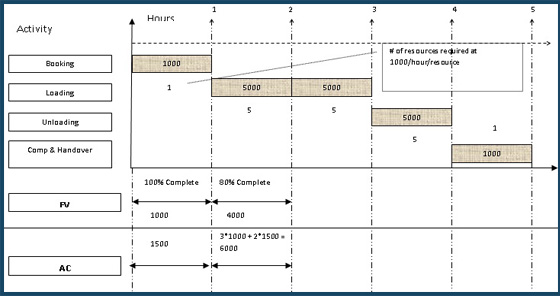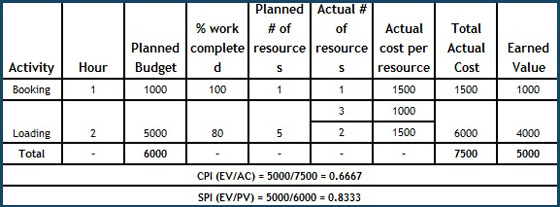When we evaluate our project performance, we need to also forecast how much more money is needed in this project and what could be the revised budget. Cost forecasts is a very important topic from PMP® exam point. Revised budget is nothing but EAC (Estimate at Completion) which will be different from initial planned numbers i.e. BAC (Budget at completion). EAC and ETC (Estimate to complete) can be calculated in different ways depending on the reason of variations. In current blog, I’m about to disclose ‘How to calculate EAC & ETC with Typical SPI & CPI trends’

Before we venture further with the discussion on how to calculate EAC & ETC with Typical SPI & CPI trends, I would recommend you to visit my blog, ‘What is EAC & ETC and What are its Variants?’ and get a clear picture of EAC and ETC.

Now let’s come to the variant typical with both SPI & CPI or as defined in the PMBOK® EAC forecast for ETC work considering both SPI and CPI factors. As per PMBOK® Guide Fifth Edition

In this forecast, the ETC work will be performed at an efficiency rate that considers both the cost and schedule performance indices. This method is most useful when the project schedule is a factor impacting the ETC effort. Variations of this method weight the CPI and SPI at different values (e.g., 80/20, 50/50, or some other ratio) according to the project manager’s judgment

In other words, this is a typical scenario impacted with both cost and schedule variations. Current variations of cost and schedule are GOING to continue at current CPI & SPI rate respectively. Project, from this point onwards, has to consider current variations to forecast the revised budget of the project

Let’s take an example and try to understand and discuss on an atypical variant of EAC and ETC. Let’s assume a Movers & Packers company took a project of shifting stuff from one place to another. The full project is planned for 5 hours as below:There are total 4 activities booking, loading, unloading and completion & handover. Booking need 1 person for the first hour and will cost 1K, loading needs 5 persons for 2 hours so planned to cost 10000, Unloading needs 5 persons for 1 hour, so planned to cost 5000 and completion and handover needs 1 person for one hour so planned to take 1000. On the basis of this we have a BAC planned to be 17000 at the end of 5th hour

Let’s access the situation at the end of the 2nd hour of work. We have observed some variation to our plan as below:The variation we faced in the above example is “labour not available”. As we are discussing the typical scenario with both CPI & SPI variations, we can say that variations in cost and schedule are going to continue at current CPI & SPI rates e.g. there is a full day local festival and labour cost as well as the duration for which they are available will be fluctuating. The rest of the work (ETC) will be done considering CPI and SPI both the rates we have. This variant is used less and there is much confusion around when this can be used. After analyzing this variant from multiple angles, I come to a conclusion that this variant is used when the question is specifying below 3 things.

1. Variation in project cost is a vital factor (this is default for typical cases) i.e. CPI is required
2. Variation in project schedule is also a vital factor to be considered in forecasts i.e. SPI is required
3. Firm completion dates are asked which is not possible without considering both the variations i.e. CPI & SPI

Let’s see the formula for this variant now

• The formula for this is same as we did for CPI (pre substitution) but SPI is also considered i.e. EAC = AC + [(BAC-EV) /CPI*SPI]
• There is more to this variant. CPI and SPI are weighed on the scale of 100. Some projects use 80/20 ratio and some other 50/50. This is purely driven by the organization. The rationale behind this ratio is the strategic goal. For example, if the organization believes in big bench strength, then they are not worried about schedule much as they can arrange man power to crash the project but the project should not go over budget. On the similar lines, for some organization on time delivery is the USP and they live up to their commitment on dates even if they have to spend from their own pocket. In that case SPI is given more importance.
• If we don’t consider any ratio or weightage for our example: EAC = 7500 + [(17000-5000)/0.6667 * 0.83333] = 29099. If we consider 80/20 for our project example: EAC = 7500 + [(17000-5000)/(0.8*CPI) * (0.2 * SPI) = 7500 +[(12000/0.13889)] = 93896
• As we know from general mathematics that the lesser the denominator more will be the value. Therefore, as we are deviating from our planned values (CPI and SPI are 1), lesser will be denominator and EAC will be more. We have this while we go beyond atypical to both typical cases. Go back and check others blogs for EAC value there

It’s very important to understand that if we are on schedule and no ratio is considered, this formula will be same as in typical case only with CPI. EAC = AC + (BAC-EV)/CPI*1 = AC + (BAC-EV)/CPI = BAC/CPI. Assume we are on schedule and on budget in that case there is no variations and therefore it was atypical case we shall automatically get the formula EAC = AC + (BAC-EV)/1*1 = AC +(BAC-EV) = Atypical formula. Further, as CPI is one = 1+ (BAC/AC –EV/AC) = 1+BAC-1 = BAC i.e. EAC = BAC if we are not having any variation

You may wonder what to do if my current variants are not typical with CPI & SPI trends? Yes, we have other ways of calculating ETC.

In a similar scenario when we observe the current variance are the typical with the CPI trend only i.e. we are going to observe similar cost overrun or under run in the coming days, we need to factor the variance in the initial budget by way of adjusting budget based on variance for remaining duration, for more details check our blogs on ‘How to Calculate EAC & ETC with Typical CPI  Trend?’

In a different scenario when we observe the current variance are the atypical i.e. current variances were one time and the rest of the work will be done at budgeted rate. We don’t need to consider variations in the rest of the work, for more details check our blog on ‘How To Calculate ETC When Variance Is Atypical?’

There could be a case where you find the initial estimate of the project having errors and these errors cannot be fixed just by taking into account the current variances. So what to do? Simple, we need to re-estimate for the remaining project duration, this variance is explained in the blog, ‘How to Calculate ETC When Initial Estimate Were Erroneous?’

By now, you must have clearly understood How to Calculate EAC & ETC with Typical SPI & CPI  Trends. For further questions and doubts on the subject, drop us a comment on our Discussion Forum. You can also log into our YouTube channel watch the videos on the related topics.

```Enroll to our FREE PMP® Introductory Program to learn more about PMP® certification
```